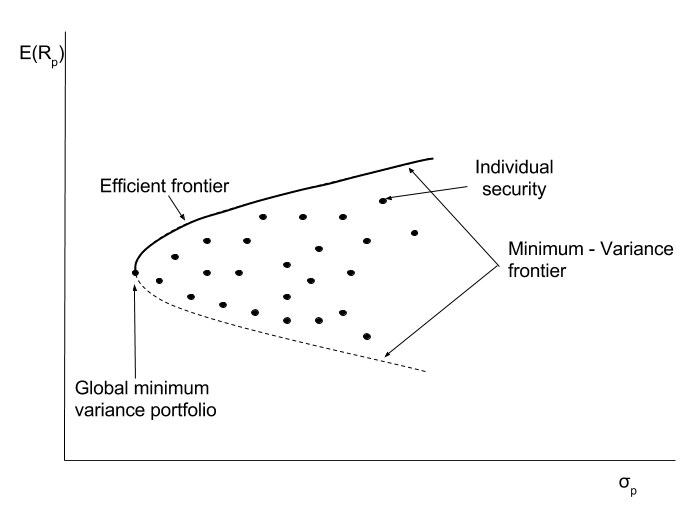Concept 65: Minimum-Variance and Efficient Frontiers | IFT World
101 Concepts for the Level I Exam

# Concept 65: Minimum-Variance and Efficient Frontiers• Investment opportunity set: Portfolios with varying weights of all the individual assets (both risky assets and risk free assets) available to the investors are plotted on a graph where return is on the y-axis and standard deviation (risk) is on the x-axis.
• For a given rate of return, there will be a portfolio with minimum variance (risk) available in the opportunity set. The curve connecting such portfolios with minimum variance is called the minimum-variance frontier.
• The portfolio having the least risk (variance) among all the portfolios of risky assets is called the global minimum-variance portfolio.
• As a risk averse investor will only select the portfolio giving higher return for a given level of risk, the part of minimum-variance frontier above the global minimum-variance portfolio is called the efficient frontier.

The graph below shows these points:Zoom Classes for Nov-23 Exam Starting Now!
This is default text for notification bar